## Blurring and Smoothing OpenCV Python Tutorial

In this OpenCV with Python tutorial, we're going to be covering how to try to eliminate noise from our filters, like simple thresholds or even a specific color filter like we had before: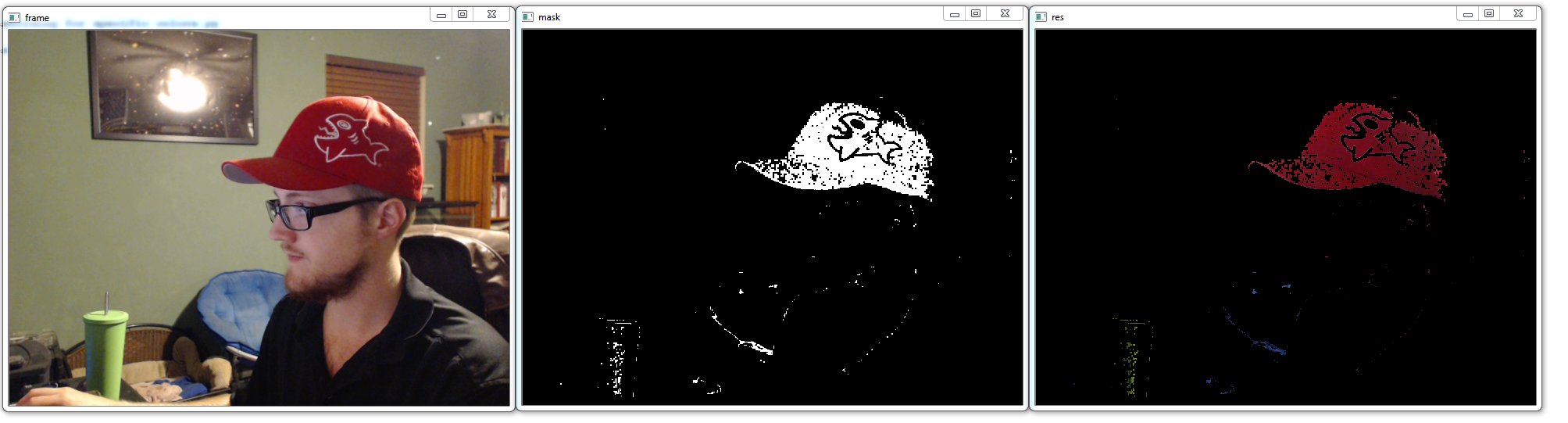As you can see, we have a lot of black dots where we'd prefer red, and a lot of other colored dots scattered about. We can use various blurring and smoothing techniques to attempt to remedy this a bit. We can start with some familiar code:

```import cv2
import numpy as np

cap = cv2.VideoCapture(0)

while(1):

hsv = cv2.cvtColor(frame, cv2.COLOR_BGR2HSV)

lower_red = np.array([30,150,50])
upper_red = np.array([255,255,180])

Now, let's apply a simple smoothing, where we do a sort of averaging per block of pixels. In our case, let's do a 15 x 15 square, which means we have 225 total pixels.

```    kernel = np.ones((15,15),np.float32)/225
smoothed = cv2.filter2D(res,-1,kernel)
cv2.imshow('Original',frame)
cv2.imshow('Averaging',smoothed)

k = cv2.waitKey(5) & 0xFF
if k == 27:
break

cv2.destroyAllWindows()
cap.release()```

Result: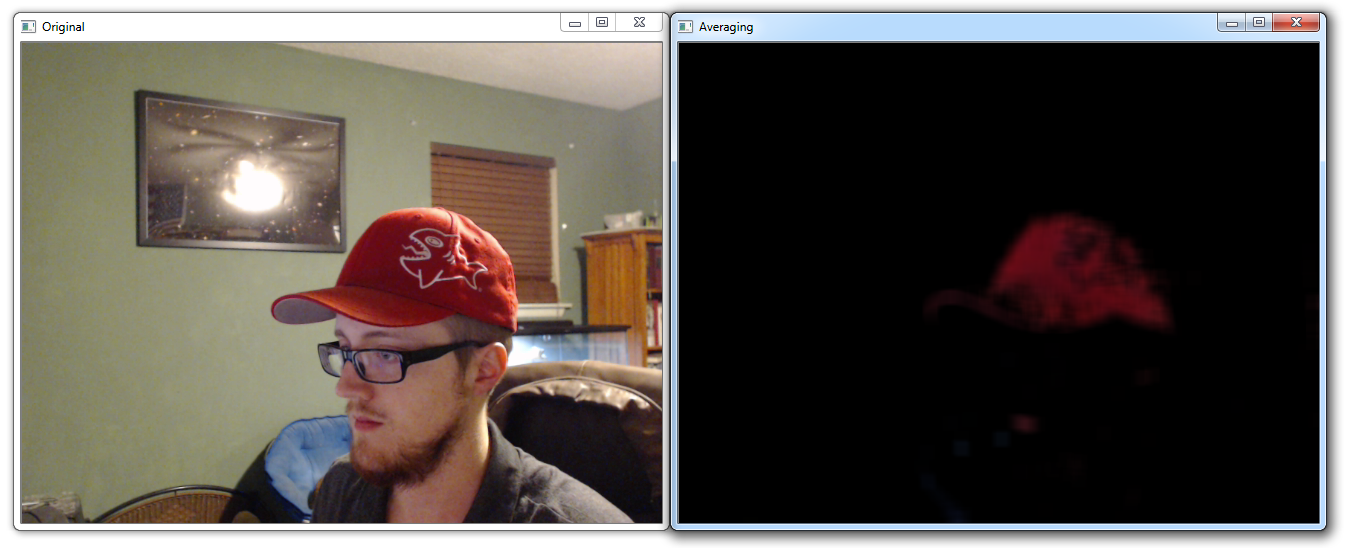This one is simple enough, but the result sacrifices a lot of granularity. Next, let's try some Gaussian Blurring:

```    blur = cv2.GaussianBlur(res,(15,15),0)
cv2.imshow('Gaussian Blurring',blur)```

Result: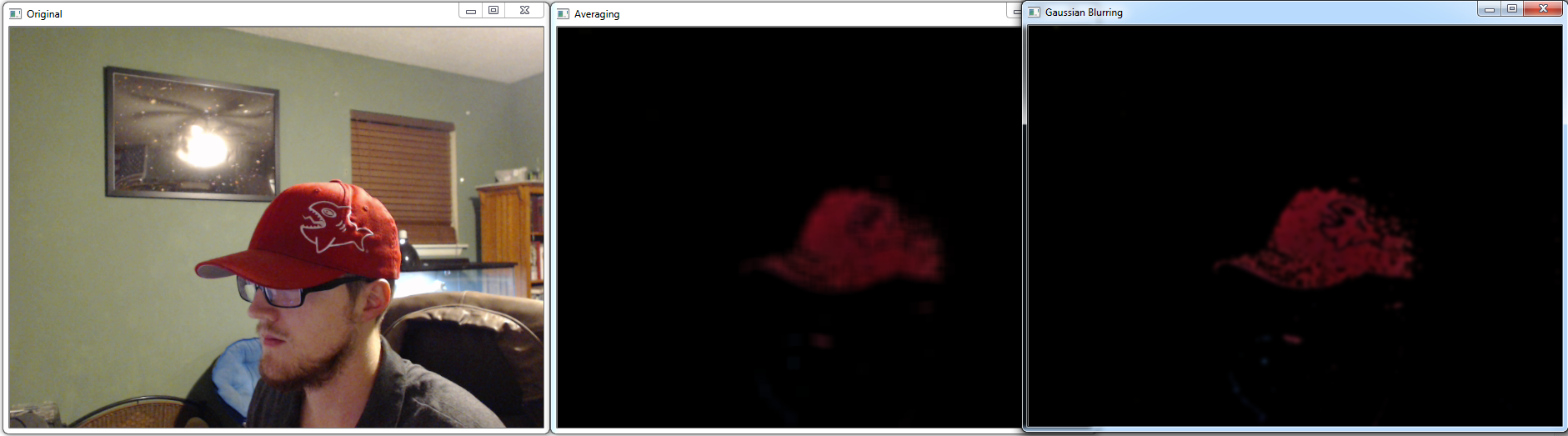Another option is what is called Median Blur:

```    median = cv2.medianBlur(res,15)
cv2.imshow('Median Blur',median)```

Result: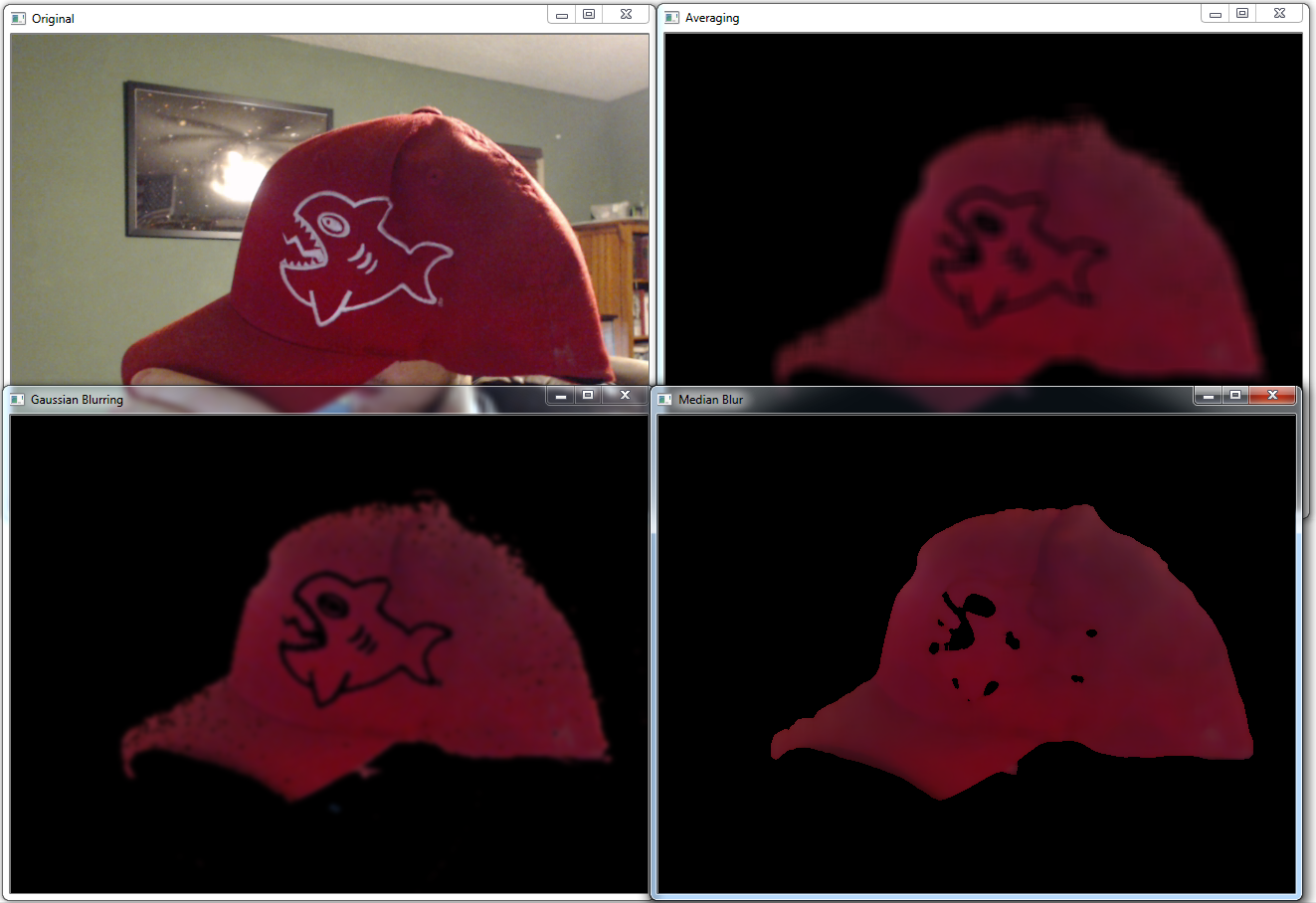Finally, another option is the bilateral blur:

```    bilateral = cv2.bilateralFilter(res,15,75,75)
cv2.imshow('bilateral Blur',bilateral)```

All of the blurs compared: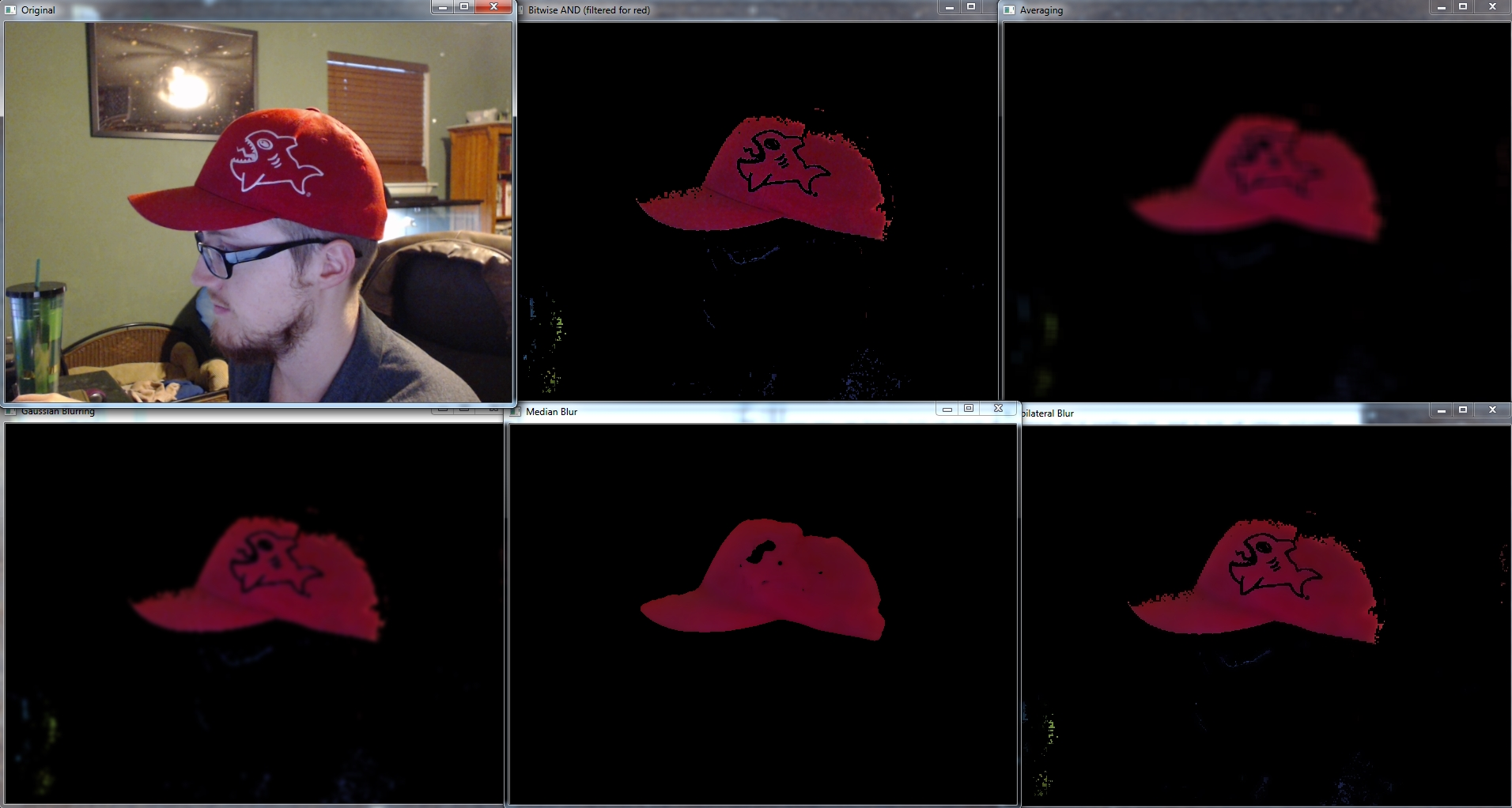At least in this case, I would probably go with the Median Blur, but different lightings, different thresholds/filters, and otherwise different goals and objectives may dictate that you use one of the others.

In the next tutorial, we're going to be discussing morphological transformations.

The next tutorial:• Drawing and Writing on Image OpenCV Python Tutorial

• Image Operations OpenCV Python Tutorial

• Image arithmetics and Logic OpenCV Python Tutorial

• Thresholding OpenCV Python Tutorial

• Color Filtering OpenCV Python Tutorial

• Blurring and Smoothing OpenCV Python Tutorial
• Morphological Transformations OpenCV Python Tutorial

• Canny Edge Detection and Gradients OpenCV Python Tutorial

• Template Matching OpenCV Python Tutorial

• GrabCut Foreground Extraction OpenCV Python Tutorial

• Corner Detection OpenCV Python Tutorial

• Feature Matching (Homography) Brute Force OpenCV Python Tutorial

• MOG Background Reduction OpenCV Python Tutorial

• Haar Cascade Object Detection Face & Eye OpenCV Python Tutorial